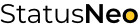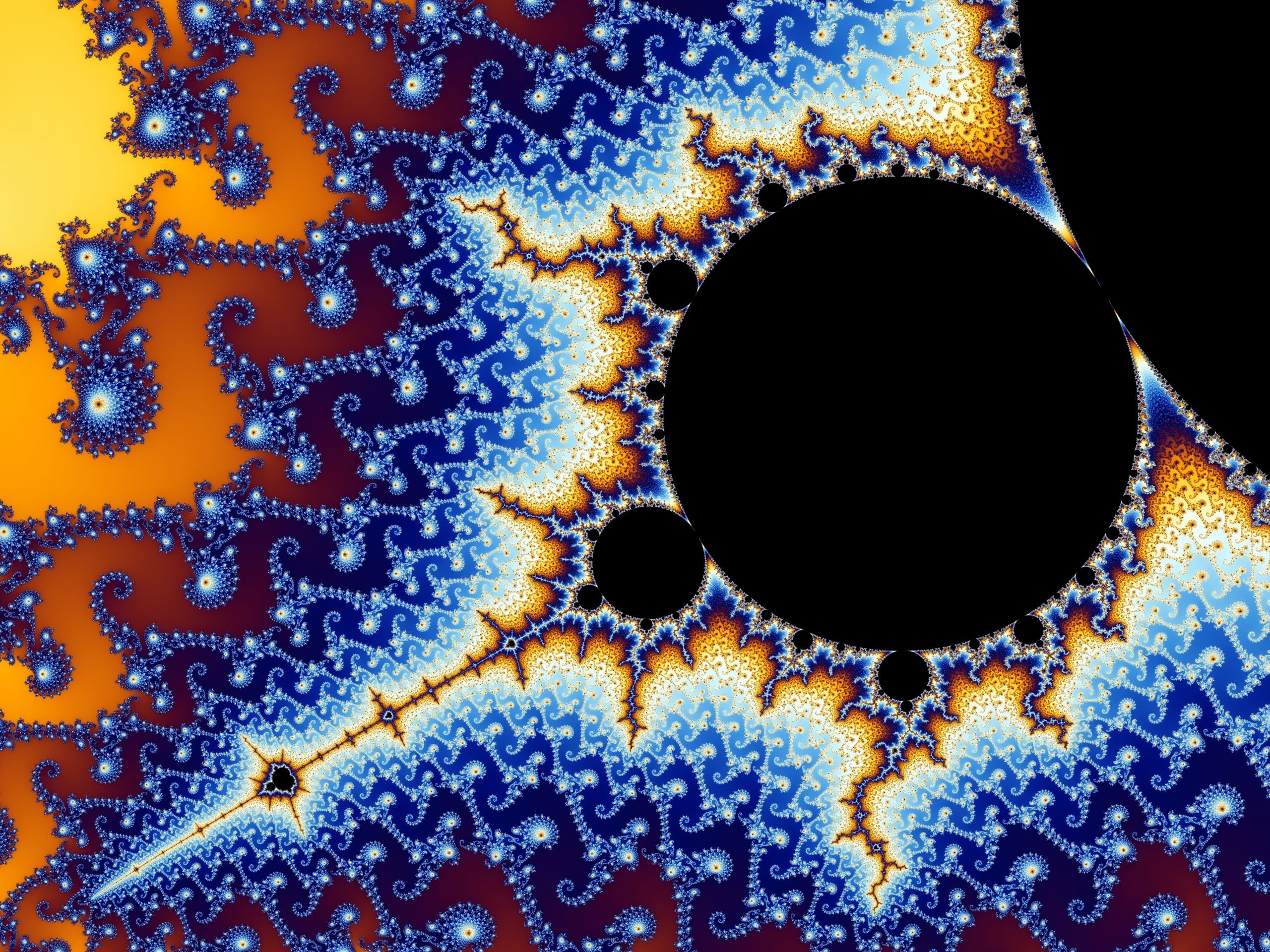WE ARE EXPERTS IN TECHNOLOGY

## Let’s Work Together# StatusNeo## Fractal Dimensions for Feature Extraction in Time Series

Time Series data exists all around us. It ranges from stock market data showing trends in real time, to healthcare data like EEG, MEG and ECG signals. Time series data is usually recorded in the temporal domain. Therefore, it has a sampling frequency. A sampling frequency of 512 Hz implies 512 values recorded in 1 second. So, for just 5 minutes of duration, there would be 153600 values in just 1 time series. Oftentimes, datasets comprise hundreds or even thousands of time series. Such a large amount of data cannot be analyzed directly. Features must be extracted in a way that preserves the characteristics of the time series and at the same time, reduce its dimensionality.

There are many popular algorithms for feature extraction from signals such as Principal Component Analysis, Auto Regressive Methods, Fourier Transforms, Wavelet Transforms, etc. However, Fractal Dimensions are often overlooked in this regard. This post discusses different methods of calculating fractal dimensions and how they can be used for feature extraction.

## What is a Fractal?

A Fractal is a curve or a geometrical structure having a characteristic recurring pattern which can be symmetric or asymmetric. They are self-similar across different scales. They are created by repeating a simple process over and over again recursively. Fractals can be interpreted as pictures representing ‘Order in chaos’. From a cursory glance they seem highly chaotic in nature and contain complexities beyond comprehension. But the process in which fractals are created is highly ordered and can be defined by laws of mathematics as shown later in this post. Few examples of Fractals are mentioned below: –

• Koch’s Snowflake – It is one of the earliest fractal curves to have been discovered. It is a a figure in the form of a snowflake. When zoomed, it keeps repeating its geometrical pattern infinitely.

• Sierpinski’s Triangle – A fractal with repeating patterns of triangles. Upon looking closely, it can be seen that every big triangle consists of 3 smaller triangles, with all having the same pattern.
• Mandelbrot – The term Mandelbrot set is used to refer both to a general class of fractal sets and to a particular instance of such a set. In general, a Mandelbrot set marks the set of points in the complex plane such that the corresponding Julia set is  connected and not computable. The Mandelbrot set is the set obtained from the quadratic recurrence equation:
•• A plot of the mandelbrot fractal looks like the following image: –

Fractal Dimensions exist naturally all around us as well. Some examples of Fractals found in nature are as follows: –

## What is a Fractal Dimension?

From a higher level, fractals seem to be extremely complex in nature. They can be symmetric and asymmetric both. The only way to understand and analyze such a geometrical figure is to quantify its complexity, which is essentially what a fractal dimension is. It is a measure of the complexity of the figure. The higher the complexity, the higher the fractal dimension.

The formula for Fractal Dimension has been derived below.

1. As seen in the image above, a straight line has only one dimension. If we take a multiplication factor (R) and Dimension (D), and multiply the length of the line by 2 (i.e R=2), we would get essentially 2 lines. Let’s assume number of outputs as N. So for R=2 and D=1, we get N=2.
2. In the 2nd Dimension (i.e D=2), we can have a square having side L. But if we multiply the length of the line by 2 (i.e R=2), we get 4 squares (i.e R^2). For R=3, we get 9 squares (i.e R^2), and so on.
3. For the 3rd Dimension (D=3), we can have a cube having side L. By multiplying the line by 2 (R=2), we get 8 cubes. For R=3, D=3, we get N=27 (i.e R^3)

Upon observing the above points, it is clearly evident, that for regular symmetrical figures, N=R^D. Upon solving further we get,

For the Koch’s curve, as seen in the image below, a key thing to be observed is that for every new order, there are 4 repeating figures from the preceding order. For example, the figure in Order=1 has 4 straight lines from the figure in Order=0. Similarly, the figure in order=2 is basically the figure from order=3 repeating 4 times. Therefore, it can be said that R=3 and N=4, for Order=3. Substituting these values in the formula, we get D=1.262 for order=3, which is the Fractal Dimension of the figure.

This repetition of figures from the preceding order can be seen in every order as we go higher, as seen in the image above. As we move up the order, the complexity of the figure increases and so does the Fractal Dimension. This proves that fact that Fractal Dimension can effectively represent the complexity of a figure. This property is applied on Time Series data to compute a value representing the complexity of the waveform as an FD. The above formula is fairly simple and is only applicable on symmetric regular structures. The different ways to calculate FDs for a time series are fairly complex in nature. A few of them are listed later in this post.

## Applications of Fractal Dimensions

• EEG/MEG Analysis: Electroencephalogram (EEG) is a non invasive device which is used to record the voltage fluctuations in the brain using electrodes attached at specific points on the skull for a particular duration of time. Usually there are 32 or 64 electrodes that are used at specific points.

Each electrode records its own time series of voltage fluctuations in a separate channel. By effectively analyzing the EEG data, diseases like Alzheimer’s, Parkinson’s, Autism, Schizophrenia and many others can be detected and diagnosed in an automated manner. There has been a huge amount of research in this domain. Many research projects have employed Fractal Dimensions to extract features from the EEG signal. Usually, the EEG signal is segmented into windows of 1 second, 5 second or any such duration, and the fractal dimension of these windows are calculated. These FDs adequately represent the complexity changes in the signal and reduce the dimensionality by a large margin. For a sampling frequency of 512Hz, 5 seconds would hold 2560 values. But by calculating FDs for every 1 second tumbling window, we would get just 5 FDs for 5 seconds as features. These FDs can then be fed into an ML/DL model for classification.

• Crack Identification in Beam structures: The presence of a crack in a structure induces a local flexibility which affects the dynamic behaviour of the whole structure to a considerable degree. It results in reduction of natural frequencies and changes in mode shapes of vibration. An analysis of these changes makes it possible to identify cracks. FDs can be used as a feature extraction method for this vibration signal analysis.
• Image Compression and Resolution: Fractal Image Coding (FIC) is an algorithm used for lossy compression of digital images. It is mainly used for textures and natural images having recurring or similar parts. FDs are also used for roughness, smoothness use cases.

## Fractal Dimension of a Time Series/Signal

There are many methods of calculating fractal dimension of a time series.

• Higuchi’s FD – It was originally formulated by T. Higuchi. Given a time series X:{1, ….. N} -> R consisting of N data points and a parameter kmax >= 2, the Higuchi Fractal Dimension (HFD) of X is calculated in the following way:
• For each k in {1, … kmax } and m in {1, …. k} the Length Lm (k) is defined by
•• The length L(k) is defined by the average value of the k lengths L1 (k), …….. Lk (k)
•• The slope of the best-fitting linear function through the below data points is defined to be the Higuchi Fractal Dimension of the time series X.
•• Petrosian FD: The Petrosian Fractal Dimension of a time series X is defined by:
•where N is the length of the time series and Ndelta is the number of sign changes in the signal derivative.
• Few other methods of calculating FD are:
• Katz FD
• Box Counting Method

Fractal Dimensions have a very diverse set of applications. They can be used for Signal Processing as well as Image Processing. They have deep mathematical foundations with some uncharted territory even to this day. These mathematical structures can be found all around us, with objects ranging from a few centimetres wide like snowflakes, to objects at molecular levels. Such a correlation of nature with mathematics can hardly be seen anywhere else and is absolutely beautiful.

References:

https://mathworld.wolfram.com/MandelbrotSet.html